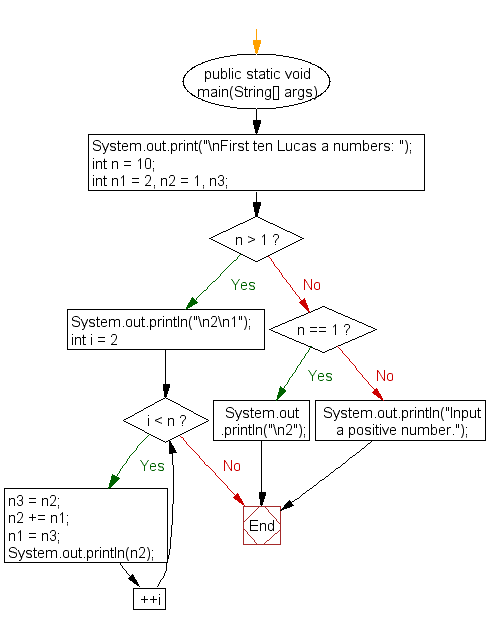﻿ Java exercises: Display first 10 lucus numbers - w3resource# Java Exercises: Display first 10 lucus numbers

## Java Numbers: Exercise-7 with Solution

Write a Java program to display first 10 lucus numbers.

The Lucas numbers or series are an integer sequence named after the mathematician François Édouard Anatole Lucas, who studied both that sequence and the closely related Fibonacci numbers. Lucas numbers and Fibonacci numbers form complementary instances of Lucas sequences.
The sequence of Lucas numbers is: 2, 1, 3, 4, 7, 11, 18, 29, ….

Sample Solution:

Java Code:

``````import java.util.Scanner;
public class Example7 {
public static void main(String[] args) {

System.out.print("\nFirst ten Lucas a numbers: ");
int n = 10;
int n1 = 2, n2 = 1, n3;
if (n > 1){
System.out.println("\n2\n1");
for(int i = 2; i < n; ++i){
n3 = n2;
n2 += n1;
n1 = n3;
System.out.println(n2);
}
}
else if (n == 1)
System.out.println("\n2");

else
System.out.println("Input a positive number.");
}
}
```
```

Sample Output:

```First ten Lucas a numbers:
2
1
3
4
7
11
18
29
47
76
```

Flowchart:Java Code Editor:

What is the difficulty level of this exercise?

﻿

## Java: Tips of the Day

Array vs ArrayLists:

The main difference between these two is that an Array is of fixed size so once you have created an Array you cannot change it but the ArrayList is not of fixed size. You can create instances of ArrayLists without specifying its size. So if you create such instances of an ArrayList without specifying its size Java will create an instance of an ArrayList of default size.

Once an ArrayList is full it re-sizes itself. In fact, an ArrayList is internally supported by an array. So when an ArrayList is resized it will slow down its performance a bit as the contents of the old Array must be copied to a new Array.

At the same time, it's compulsory to specify the size of an Array directly or indirectly while creating it. And also Arrays can store both primitives and objects while ArrayLists only can store objects.

Ref: https://bit.ly/3o8L2KH